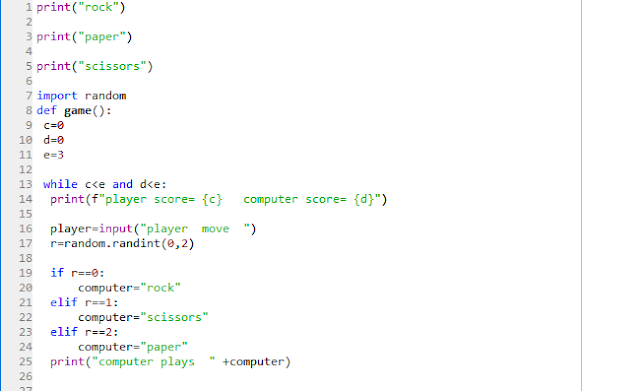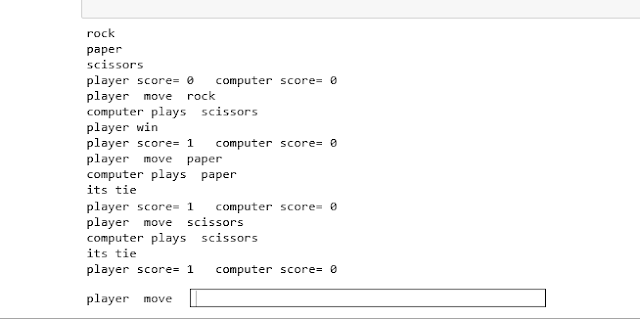# Python Programming: ROCK, PAPER, SCISSOR Game Code

Python Programming: ROCK, PAPER, SCISSOR your First Python Game Code.

Python is A general-purpose high-level programming language.

ROCK, PAPER, SCISSOR - a basic level game for beginners to code.winning rule for the game
ROCK ROCK > SCISSOR
PAPER
CODE:

print("rock")

print("paper")

print("scissors")

import random
def game():
c=0
d=0
e=3

while c   print(f"player score= {c}   computer score= {d}")

player=input("player  move  ")
r=random.randint(0,2)

if r==0:
computer="rock"
elif r==1:
computer="scissors"
elif r==2:
computer="paper"
print("computer plays  " +computer)

if player=="rock" and computer=="scissors":

print("player win")
c=c+1

elif player=="rock" and computer=="paper":
print("computer win")
d=d+1

elif player=="paper" and computer=="scissors":
print("computer win")
d=d+1

elif computer=="rock" and player=="scissors":
print("computer win")
d=d+1

elif computer=="rock" and player=="paper":
print("player win")
c=c+1

elif computer=="paper" and player=="scissors":
print("player win")
c=c+1

elif player==computer:
print("its tie")

else:
print("something went wrong")
if c==3:
print(f"your final score:{c} computer final score:{d}")
print("Game over : you win")
s=input("play again(Y)?")

if s=="y":
print("welcome again")
game()

else:
print("thanku")
break

elif d==3:
print(f"your final score:{c} computer final score:{d}")
print("Game over :computer win")
s=input("play again(Y)?")
if s=="y":
print("welcome again")
game()

else:
print("thanku")
break
game()

OUTPUTalso read Python: A complete overview in 5 min

1.